# Simulating Chandra ACIS-S Spectra with Sherpa## Overview

#### Synopsis:

This thread illustrates the use of the Sherpa fake_pha command to simulate a spectrum of a point source obtained with the ACIS-S detector aboard Chandra, with and without consideration of a background component.

If you do not have experience with simulating X-ray spectral data in Sherpa, you may wish to follow the "step-by-step" example in the introductory simulation thread, before attempting the sample analysis in this thread.

Last Update: 12 Dec 2022 - updated for CY 25/CIAO 4.15, no content change.

## Contents

In order to simulate a Chandra ACIS-S spectrum with Sherpa, we must define an instrument response with the appropriate ARF (auxiliary response function) and RMF (redistribution matrix function) files. These files may be downloaded from the Chandra Proposal Planning page of the CalDB (Calibration Database) website, where sample RMFs and corresponding ARFs positioned at the aimpoint of the ACIS-S array (and at selected off-axis points) are available.

In this thread, we use the files aciss_aimpt_cy25.arf and aciss_aimpt_cy25.rmf, positioned at the default pointing for ACIS-S.

The Sherpa fake_pha command calculates a 1-D spectral data set based on a defined instrument response and source model expression. For extensive details on the fake_pha functionality, see the introductory simulation thread Simulating X-ray Spectral Data (PHA): the fake_pha command.

To learn how to simulate the spectrum of a point source which includes a background component, follow the second half of this thread, "Including a background component".

## Defining the Instrument Response

We begin by establishing the instrument response corresponding to the default pointing of the ACIS-S detector:

sherpa> arf1 = unpack_arf("aciss_aimpt_cy25.arf")

sherpa> print(arf1)
name     = aciss_aimpt_cy25.arf
energ_lo = Float64
energ_hi = Float64
specresp = Float64
bin_lo   = None
bin_hi   = None
exposure = 8037.3177424371
ethresh  = 1e-10

sherpa> rmf1 = unpack_rmf("aciss_aimpt_cy25.rmf")
name     = aciss_aimpt_cy25.rmf
energ_lo = Float64
energ_hi = Float64
n_grp    = UInt64
f_chan   = UInt32
n_chan   = UInt32
matrix   = Float64
e_min    = Float64
e_max    = Float64
detchans = 1024
offset   = 1
ethresh  = 1e-10


Here, the ARF and RMF data are loaded and assigned to a set of variables with the unpack_* commands. These variables will be used to assign the instrument response to the faked data set we will create in the next section, "Defining a Source Model Expression".

## Defining a Source Model Expression

Now that we have loaded the ARF and RMF instrument responses, we use the set_source command to establish a source model expression and name the faked data set which will be produced in our simulation.

sherpa> set_source(1, xsphabs.abs1 * powlaw1d.m1)
sherpa> m1.gamma = 2
sherpa> abs1.nh = 0.2


We have defined a source model expression for this simulation using an absorbed 1-D power-law model with a Galactic neutral hydrogen column density of 2×1021 cm-2 and a power-law photon index of 2.

## Running the Simulation with fake_pha

Simulating the Chandra spectrum means taking the defined model expression, folding it through the Chandra ACIS-S response, and applying Poisson noise to the counts predicted by the model. The simulation is run with fake_pha, which has four required arguments: dataset ID, ARF, RMF, and exposure time. We decide to simulate an ACIS-S spectrum resulting from a 50 ks exposure of a point source.

sherpa> fake_pha(1, arf1, rmf1, exposure=50000, grouped=False, backscal=1.0)


This command associates the data ID 1 with a simulated data set based on the assumed exposure time, instrument response, and source model expression we defined earlier; Poisson noise is added to the modeled data.

Note that as of Sherpa in CIAO 4.2, the 'arf' and 'rmf' arguments of the fake_pha command can accept filenames directly; e.g., we could have done the following:

sherpa> fake_pha(1, arf="aciss_aimpt_cy25.arf", rmf="aciss_aimpt_cy25.rmf", exposure=50000, grouped=False, backscal=1.0)


For detailed information on the available options for setting the 'arf' and 'rmf' arguments of fake_pha, refer to the fake_pha ahelp file.

We may inspect some basic properties of the new data set with the show_data command:

sherpa> show_data()
Data Set: 1
Filter: 0.0073-14.9504 Energy (keV)
Noticed Channels: 1-1024
name           = faked
channel        = Int64
counts         = Float64
staterror      = None
syserror       = None
bin_lo         = None
bin_hi         = None
grouping       = None
quality        = None
exposure       = 50000
backscal       = 1.0
areascal       = None
grouped        = False
subtracted     = False
units          = energy
rate           = True
plot_fac       = 0
response_ids   = 
background_ids = []

RMF Data Set: 1:1
name     = aciss_aimpt_cy25.rmf
energ_lo = Float64
energ_hi = Float64
n_grp    = UInt64
f_chan   = UInt32
n_chan   = UInt32
matrix   = Float64
e_min    = Float64
e_max    = Float64
detchans = 1024
offset   = 1
ethresh  = 1e-10

ARF Data Set: 1:1
name     = aciss_aimpt_cy25.arf
energ_lo = Float64
energ_hi = Float64
specresp = Float64
bin_lo   = None
bin_hi   = None
exposure = 8037.3177424371
ethresh  = 1e-10


Note that the simulated data set currently does not have the correct normalization—the flux of the simulated data is incorrect because the default power-law normalization is arbitrarily set to 1.0, as shown with the show_model command:

sherpa> show_model()
Model: 1
apply_rmf(apply_arf((50000 * (xsphabs.abs1 * powlaw1d.m1))))
Param        Type          Value          Min          Max      Units
-----        ----          -----          ---          ---      -----
abs1.nh      thawed          0.2            0       100000 10^22 atoms / cm^2
m1.gamma     thawed            2          -10           10
m1.ref       frozen            1 -3.40282e+38  3.40282e+38
m1.ampl      thawed            1            0  3.40282e+38


To correct the flux we need to adjust the normalization, as demonstrated in the section "Defining the Model Normalization for the Simulated Data".

## Defining the Model Normalization for the Simulated Data

Before we can use the simulated data set for scientific analysis, it must be re-normalized to match the flux (or total counts) required by our selected source.

The 0.2-10 keV flux in the simulated spectrum is 3.89×10-9 ergs cm-2 s-1:

sherpa> calc_energy_flux(0.2,10,1)
3.894440207498213e-09


The 0.2-10 keV flux of a source in our Chandra proposal, for example, has been measured at 1.0×10-12 ergs cm-2 s-1. Therefore, the correct normalization is $$\frac{1.\mathrm{e}-12}{3.894440207498213\mathrm{e}-09}=0.00025677631359563216$$:

sherpa> my_flux = 1.e-12

sherpa> norm = my_flux / calc_energy_flux(0.2, 10, 1)

sherpa> print(norm)
0.00025677631359563216

sherpa> set_par(m1.ampl, norm)

sherpa> show_model(1)
Model: 1
apply_rmf(apply_arf((50000.0 * (xsphabs.abs1 * powlaw1d.m1))))
Param        Type          Value          Min          Max      Units
-----        ----          -----          ---          ---      -----
abs1.nH      thawed          0.2            0        1e+06 10^22 atoms / cm^2
m1.gamma     thawed            2          -10           10
m1.ref       frozen            1 -3.40282e+38  3.40282e+38
m1.ampl      thawed  0.000256776            0  3.40282e+38

sherpa> fake_pha(1, arf1 ,rmf1, exposure=50000)

sherpa> prefs = get_data_plot_prefs()

sherpa> prefs["yerrorbars"] = False      # remove y-error bars from plot

sherpa> plot_data(1)



With the new normalization, the simulated flux is correctly set at the measured flux of 1×10-12 ergs cm-2 s-1. A plot of the data is shown in Figure 1.

### Figure 1: Plot of simulated source spectrum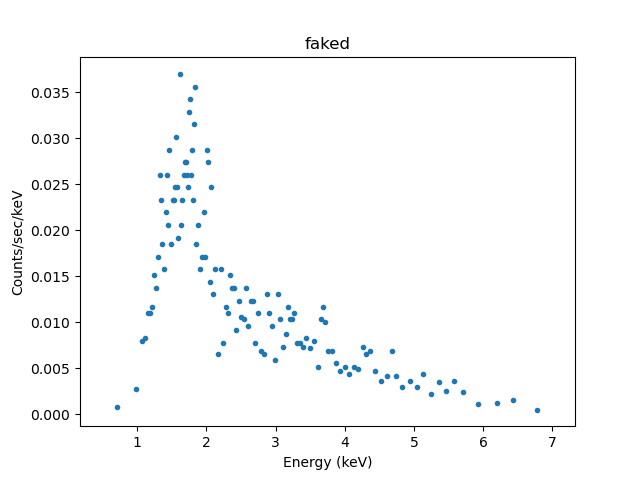### Figure 1: Plot of simulated source spectrum

Note that we could have chosen to re-normalize the simulated data set to match the required total counts instead of flux. For example:

sherpa> my_counts = 10000

sherpa> norm_counts = my_counts / calc_data_sum(0.5, 8., 1)

sherpa> print(norm_counts)
3.459010722933241


## Writing the Simulated Data to Output Files

We may use the save_pha command to write the simulated data as a PHA file, with a header containing the exposure time value and paths to the ARF and RMF files:

sherpa> save_pha(1, "simulation1.pha")


We also have the option to save the data to a FITS or ASCII table file with the save_arrays command:

sherpa> save_arrays("my_sim_data.fits", [get_model_plot(1).xlo, get_model_plot(1).y], ascii=False)

sherpa> save_arrays("my_sim_data.txt", [get_model_plot(1).xlo, get_model_plot(1).y], ascii=True)


## Fitting the Simulated Data

The simulated data set may be filtered and fit as any other data set in Sherpa. For example, we can choose to filter the simulated data to include only the counts in a restricted energy range, such as 0.5 keV-7.0 keV:

sherpa> calc_energy_flux(0.2, 10, 1)  # ergs cm-2 s-1
1e-12

sherpa> calc_energy_flux(0.5, 7, 1)
8.358234861554698e-13

sherpa> calc_data_sum(0.5, 7, 1)  # counts
2874.0

sherpa> notice(0.5, 7)
dataset 1: 0.0073:14.9504 -> 0.4964:7.008 Energy (keV)

sherpa> show_filter()
Data Set Filter: 1
0.4964-7.0080 Energy (keV)


Then, we can fit the simulated data set with the source model expression we used to create it:

sherpa> set_method("neldermead")
sherpa> set_stat("cstat")

sherpa> fit()
Dataset               = 1
Statistic             = cstat
Initial fit statistic = 492.451
Final fit statistic   = 491.728 at function evaluation 454
Data points           = 446
Degrees of freedom    = 443
Probability [Q-value] = 0.0544883
Reduced statistic     = 1.11
Change in statistic   = 0.722452
abs1.nH        0.175512
m1.gamma       1.99289
m1.ampl        0.0002537

sherpa> plot_fit(1)
WARNING: unable to calculate errors using current statistic: cstat


The resulting plot is shown in Figure 2.

### Figure 2: Plot of fit to simulated source spectrum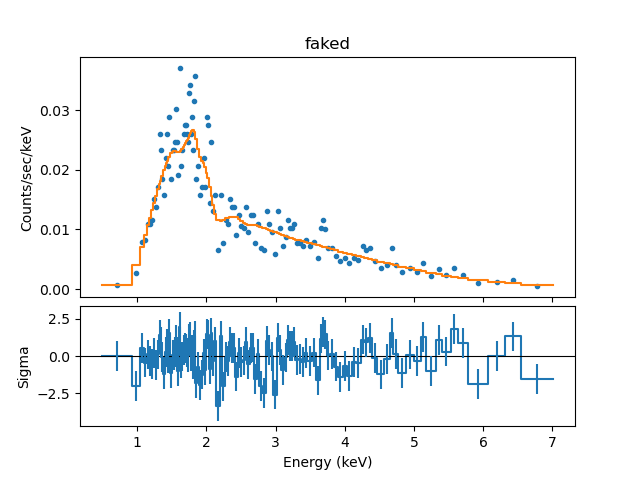### Figure 2: Plot of fit to simulated source spectrum

Next, we examine the quality of the fit with the confidence command (conf), and print the fit and confidence results with show_fit and get_conf_results, respectively.

sherpa> conf()
abs1.nH lower bound:  -0.0514072
abs1.nH upper bound:    0.0526572
m1.gamma lower bound:   -0.0713268
m1.ampl lower bound:    -2.11601e-05
m1.gamma upper bound:   0.0725769
m1.ampl upper bound:    2.32414e-05
Dataset               = 1
Confidence Method     = confidence
Iterative Fit Method  = None
Statistic             = cstat
confidence 1-sigma (68.2689%) bounds:
Param            Best-Fit  Lower Bound  Upper Bound
-----            --------  -----------  -----------
abs1.nH          0.175512   -0.0514072    0.0526572
m1.gamma          1.99289   -0.0713268    0.0725769
m1.ampl         0.0002537 -2.11601e-05  2.32414e-05

sherpa> show_fit()
name         = simplex
ftol         = 1.1920928955078125e-07
maxfev       = None
initsimplex  = 0
finalsimplex = 9
step         = None
verbose      = 0
reflect      = True

Statistic: CStat
Maximum likelihood function (XSPEC style).

This is equivalent to the XSpec implementation of the
Cash statistic _ except that it requires a model to be fit
to the background. To handle the background in the same manner
as XSpec, use the WStat statistic.

Counts are sampled from the Poisson distribution, and so the best
way to assess the quality of model fits is to use the product of
individual Poisson probabilities computed in each bin i, or the
likelihood L:

L = (product)_i [ M(i)^D(i)/D(i)! ] * exp[-M(i)]

where M(i) = S(i) + B(i) is the sum of source and background model
amplitudes, and D(i) is the number of observed counts, in bin i.

The cstat statistic is derived by (1) taking the logarithm of the
likelihood function, (2) changing its sign, (3) dropping the
factorial term (which remains constant during fits to the same
dataset), (4) adding an extra data-dependent term (this is what
makes it different to Cash, and (5) multiplying by two:

C = 2 * (sum)_i [ M(i) - D(i) + D(i)*[log D(i) - log M(i)] ]

The factor of two exists so that the change in the cstat statistic
from one model fit to the next, (Delta)C, is distributed
approximately as (Delta)chi-square when the number of counts in
each bin is high.  One can then in principle use (Delta)C instead
of (Delta)chi-square in certain model comparison tests. However,
unlike chi-square, the cstat statistic may be used regardless of
the number of counts in each bin.

The inclusion of the data term in the expression means that,
unlike the Cash statistic, one can assign an approximate
goodness-of-fit measure to a given value of the cstat statistic,
i.e. the observed statistic, divided by the number of degrees of
freedom, should be of order 1 for good fits.

Notes
-----
The background should not be subtracted from the data when this
statistic is used.  It should be modeled simultaneously with the
source.

The cstat statistic function evaluates the logarithm of each data
point. If the number of counts is zero or negative, it's not
possible to take the log of that number. The behavior in this case
is controlled by the truncate and trunc_value settings in the
.sherpa.rc file:

- if truncate is True (the default value), then
log(trunc_value) is used whenever the data value is <= 0.  The
default is trunc_value=1.0e-25.

- when truncate is False an error is raised.

References
----------

..  The description of the Cash statistic (cstat) in

Fit:Dataset               = 1
Statistic             = cstat
Initial fit statistic = 492.451
Final fit statistic   = 491.728 at function evaluation 454
Data points           = 446
Degrees of freedom    = 443
Probability [Q-value] = 0.0544883
Reduced statistic     = 1.11
Change in statistic   = 0.722452
abs1.nH        0.175512
m1.gamma       1.99289
m1.ampl        0.0002537

sherpa> print(get_conf_results())
datasets    = (1,)
methodname  = confidence
iterfitname = none
statname    = cstat
sigma       = 1
percent     = 68.26894921370858
parnames    = ('abs1.nH', 'm1.gamma', 'm1.ampl')
parvals     = (0.17551179106542386, 1.992888391856171, 0.00025370000089507306)
parmins     = (-0.051407179430835614, -0.07132679323601243, -2.116007766115048e-05)
parmaxes    = (0.052657189892153566, 0.07257691202141747, 2.324139677536204e-05)
nfits       = 76


Note that the Cstat statistic is appropriate for fitting low-counts data, but it does not calculate errors for the data points. We can group the data so that each bin contains a specified minimum number of counts, and then change the fit statistic to something more suitable to calculate the errors. Finally, we can view the results of the new fit with the plot_fit_delchi command:

sherpa> group_counts(1, 15)

sherpa> set_stat("chi2xspecvar")

sherpa> plot_fit_delchi(1)


The new fit to the grouped simulated spectrum, along with the residuals divided by the uncertainties, is shown in Figure 3.

### Figure 3: Plot of fit to simulated source spectrum, with residuals### Figure 3: Plot of fit to simulated source spectrum, with residuals

The plot may be saved as a postscript file with the Matplotlib savefig command:

sherpa> plt.savefig('simulation_fit.ps')


## Including a Background Component

In this section, we repeat the steps above to simulate a source PHA data set, but this time, including a background component. This involves adding new Sherpa commands along the way to define settings for the background data.

### Defining the Instrument Response

As before, we begin by establishing the instrument response corresponding to the default pointing of the ACIS-S detector, for both a source and background component:

sherpa> arf1 = unpack_arf("aciss_aimpt_cy25.arf")
sherpa> rmf1 = unpack_rmf("aciss_aimpt_cy25.rmf")

sherpa> bkg1_arf = arf1
sherpa> bkg1_rmf = rmf1


The source ARF and RMF data are loaded and assigned to a set of variables with the unpack_* commands. These variables will be used to assign the instrument response to both the source and background components of the faked data set we will create in the next section, "Defining Source Model Expressions → with a background component". If the background response is different than the source response, we load the appropriate background ARF and RMF files accordingly:

sherpa> bkg1_rmf = unpack_rmf("background.rmf") # separate background response
sherpa> bkg1_arf = unpack_arf("background.arf")


### Defining Source and Background Model Expressions

We define both the source and background model expressions for our simulation with set_source command, as follows:

sherpa> clean() # clear models

sherpa> set_source(1, xsphabs.abs1 * powlaw1d.m1)
sherpa> m1.gamma = 2
sherpa> abs1.nh = 0.2

sherpa> set_source("faked_bkg",  polynom1d.bkgA)
sherpa> bkgA.c0 = 1.


For the source simulation, we use an absorbed 1-D power-law model with a Galactic neutral hydrogen column density of 2×1021 cm-2 and a photon index of 2. For the background simulation, we assume a flat profile with a 1-D polynomial function.

### Running the Simulation with fake_pha

Here we run an additional fake_pha simulation for the background data set:

sherpa> fake_pha(1, arf1, rmf1, exposure=50000, grouped=False, backscal=1.0)

sherpa> fake_pha("faked_bkg", bkg1_arf, bkg1_rmf, exposure=50000, grouped=False, backscal=1.0)


These commands associate the data IDs 1 and "faked_bkg" with simulated source and background data sets, respectively, based on the assumed exposure times, instrument responses, and model expressions defined earlier; Poisson noise is added to the modeled data.

Now, we assign the "faked_bkg" data set as the background component of the faked source data set 1, using the set_bkg command.

sherpa> set_bkg(1, get_data("faked_bkg"))


We may inspect some basic properties of the new simulated data sets with the show_data command:

sherpa> show_data()
Data Set: 1
Filter: 0.0073-14.9504 Energy (keV)
Bkg Scale: 1
Noticed Channels: 1-1024
name           = faked
channel        = Int64
counts         = Float64
staterror      = None
syserror       = None
bin_lo         = None
bin_hi         = None
grouping       = None
quality        = None
exposure       = 50000
backscal       = 1.0
areascal       = None
grouped        = False
subtracted     = False
units          = energy
rate           = True
plot_fac       = 0
response_ids   = 
background_ids = 

RMF Data Set: 1:1
name     = aciss_aimpt_cy25.rmf
energ_lo = Float64
energ_hi = Float64
n_grp    = UInt64
f_chan   = UInt32
n_chan   = UInt32
matrix   = Float64
e_min    = Float64
e_max    = Float64
detchans = 1024
offset   = 1
ethresh  = 1e-10

ARF Data Set: 1:1
name     = aciss_aimpt_cy25.arf
energ_lo = Float64
energ_hi = Float64
specresp = Float64
bin_lo   = None
bin_hi   = None
exposure = 8037.3177424371
ethresh  = 1e-10

Background Data Set: 1:1
Filter: 0.0073-14.9504 Energy (keV)
Noticed Channels: 1-1024
name           = faked
channel        = Int64
counts         = Float64
staterror      = None
syserror       = None
bin_lo         = None
bin_hi         = None
grouping       = None
quality        = None
exposure       = 50000
backscal       = 1.0
areascal       = None
grouped        = False
subtracted     = False
units          = energy
rate           = True
plot_fac       = 0
response_ids   = 
background_ids = []

Background RMF Data Set: 1:1
name     = aciss_aimpt_cy25.rmf
energ_lo = Float64
energ_hi = Float64
n_grp    = UInt64
f_chan   = UInt32
n_chan   = UInt32
matrix   = Float64
e_min    = Float64
e_max    = Float64
detchans = 1024
offset   = 1
ethresh  = 1e-10

Background ARF Data Set: 1:1
name     = aciss_aimpt_cy25.arf
energ_lo = Float64
energ_hi = Float64
specresp = Float64
bin_lo   = None
bin_hi   = None
exposure = 8037.3177424371
ethresh  = 1e-10

Data Set: faked_bkg
Filter: 0.0073-14.9504 Energy (keV)
Noticed Channels: 1-1024
name           = faked
channel        = Int64
counts         = Float64
staterror      = None
syserror       = None
bin_lo         = None
bin_hi         = None
grouping       = None
quality        = None
exposure       = 50000
backscal       = 1.0
areascal       = None
grouped        = False
subtracted     = False
units          = energy
rate           = True
plot_fac       = 0
response_ids   = 
background_ids = []

RMF Data Set: faked_bkg:1
name     = aciss_aimpt_cy25.rmf
energ_lo = Float64
energ_hi = Float64
n_grp    = UInt64
f_chan   = UInt32
n_chan   = UInt32
matrix   = Float64
e_min    = Float64
e_max    = Float64
detchans = 1024
offset   = 1
ethresh  = 1e-10

ARF Data Set: faked_bkg:1
name     = aciss_aimpt_cy25.arf
energ_lo = Float64
energ_hi = Float64
specresp = Float64
bin_lo   = None
bin_hi   = None
exposure = 8037.3177424371
ethresh  = 1e-10


In the next section, we will correct the normalization of the simulated source and background data sets.

### Defining the Model Normalization for the Simulation

We determine the normalization for the background data set in the same way as with the source data set, except we use a measure of total counts instead of flux to specify that we want 200 counts in the background simulation:

sherpa> my_flux = 1.e-12
sherpa> norm = my_flux / calc_energy_flux(0.2, 10, id=1)
sherpa> print(norm)
0.00025677631359563216

sherpa> bkg_counts = 200
sherpa> bkg_norm = bkg_counts / calc_data_sum(0.2, 10., id="faked_bkg")
sherpa> print(bkg_norm)
2.0949381559591793e-06


Now we apply the calculated values to the amplitude parameters of each model, and re-evaluate the simulated data sets with the desired normalization using fake_pha:

sherpa> set_par(m1.ampl, norm)
sherpa> set_par(bkgA.c0, bkg_norm)

sherpa> show_model()
Model: 1
apply_rmf(apply_arf((50000.0 * (xsphabs.abs1 * powlaw1d.m1))))
Param        Type          Value          Min          Max      Units
-----        ----          -----          ---          ---      -----
abs1.nH      thawed          0.2            0        1e+06 10^22 atoms / cm^2
m1.gamma     thawed            2          -10           10
m1.ref       frozen            1 -3.40282e+38  3.40282e+38
m1.ampl      thawed  0.000256776            0  3.40282e+38

Model: faked_bkg
apply_rmf(apply_arf((50000.0 * polynom1d.bkgA)))
Param        Type          Value          Min          Max      Units
-----        ----          -----          ---          ---      -----
bkgA.c0      thawed  2.09494e-06 -3.40282e+38  3.40282e+38
bkgA.c1      frozen            0 -3.40282e+38  3.40282e+38
bkgA.c2      frozen            0 -3.40282e+38  3.40282e+38
bkgA.c3      frozen            0 -3.40282e+38  3.40282e+38
bkgA.c4      frozen            0 -3.40282e+38  3.40282e+38
bkgA.c5      frozen            0 -3.40282e+38  3.40282e+38
bkgA.c6      frozen            0 -3.40282e+38  3.40282e+38
bkgA.c7      frozen            0 -3.40282e+38  3.40282e+38
bkgA.c8      frozen            0 -3.40282e+38  3.40282e+38
bkgA.offset  frozen            0 -3.40282e+38  3.40282e+38

sherpa> fake_pha(1,arf1,rmf1,exposure=50000,backscal=1.0)

sherpa> fake_pha("faked_bkg",bkg1_arf,bkg1_rmf,50000,backscal=1.0)


Finally, we re-assign background data set "faked_bkg" as the background component of source data set 1 with set_bkg, to produce a single source-plus-background simulated data set:

sherpa> set_bkg(1, get_data("faked_bkg"))
sherpa> prefs = get_data_plot_prefs()
sherpa> prefs["yerrorbars"] = False      # remove y-error bars from plot
sherpa> plot_data(1)


The resulting plot is shown in Figure 4.

### Figure 4: Plot of simulated source-plus-background spectrum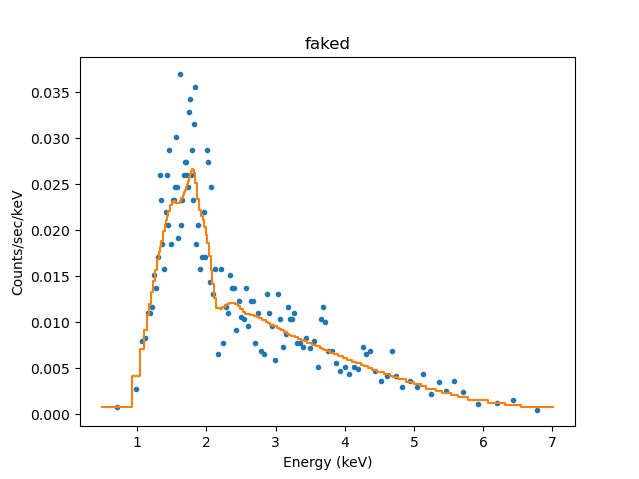### Fitting the Simulated Data

The simulated source-plus-background data set (1) is filtered to include only the counts in the energy range 0.5 keV-7.0 keV (recalling that data set 1 now contains the background information stored in data set "faked_bkg"; "faked_bkg" is no longer needed in the context of this thread).

sherpa> notice(0.5, 7)
dataset 1: 0.0073:14.9504 -> 0.4964:7.008 Energy (keV)
dataset faked_bkg: 0.4964:7.008 Energy (keV) (unchanged)

sherpa> show_filter(1)
Data Set Filter: 1
0.4964-7.0080 Energy (keV)

Data Set Filter: faked_bkg
0.4964-7.0080 Energy (keV)


Next, we fit the simulated source data with the source model expression we used to create it, and use the set_bkg_model command to incorporate the background model into the fit:

sherpa> set_bkg_model(1, bkgA, 1)  # set model for bkg_id=1 of data set id=1

sherpa> set_stat("cstat")

sherpa> fit(1)
Dataset               = 1
Statistic             = cstat
Initial fit statistic = 970.474
Final fit statistic   = 936.078 at function evaluation 4086
Data points           = 892
Degrees of freedom    = 888
Probability [Q-value] = 0.127845
Reduced statistic     = 1.05414
Change in statistic   = 34.3952
abs1.nH        0.292181
m1.gamma       2.22134
m1.ampl        0.000294912
bkgA.c0        1.89788e-06

sherpa> plot_fit(1)
WARNING: unable to calculate errors using current statistic: cstat


The resulting plot is shown in Figure 5.

### Figure 5: Plot of fit to simulated source-plus-background spectrum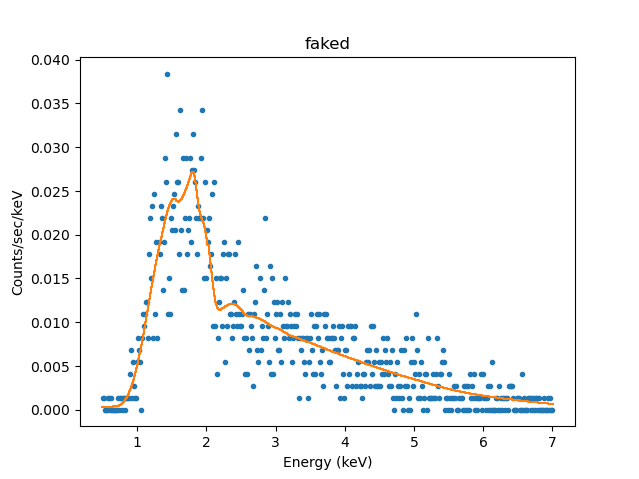### Figure 5: Plot of fit to simulated source-plus-background spectrum

Now we can examine the quality of the fit with the confidence command (conf), and return the fit and confidence results with show_fit and get_conf_results, respectively.

sherpa> conf(1)
abs1.nH lower bound:  -0.0610468
abs1.nH upper bound:    0.0622968
m1.gamma lower bound:   -0.0865563
bkgA.c0 lower bound:    -1.40684e-07
m1.ampl lower bound:    -2.85371e-05
m1.gamma upper bound:   0.0884315
m1.ampl upper bound:    3.20748e-05
bkgA.c0 upper bound:    1.47437e-07
Dataset               = 1
Confidence Method     = confidence
Iterative Fit Method  = None
Statistic             = cstat
confidence 1-sigma (68.2689%) bounds:
Param            Best-Fit  Lower Bound  Upper Bound
-----            --------  -----------  -----------
abs1.nH          0.292181   -0.0610468    0.0622968
m1.gamma          2.22134   -0.0865563    0.0884315
m1.ampl       0.000294912 -2.85371e-05  3.20748e-05
bkgA.c0       1.89788e-06 -1.40684e-07  1.47437e-07

sherpa> print(show_fit())
name         = simplex
ftol         = 1.1920928955078125e-07
maxfev       = None
initsimplex  = 0
finalsimplex = 9
step         = None
verbose      = 0
reflect      = True

Statistic: CStat
Maximum likelihood function (XSPEC style).

This is equivalent to the XSpec implementation of the
Cash statistic _ except that it requires a model to be fit
to the background. To handle the background in the same manner
as XSpec, use the WStat statistic.

Counts are sampled from the Poisson distribution, and so the best
way to assess the quality of model fits is to use the product of
individual Poisson probabilities computed in each bin i, or the
likelihood L:

L = (product)_i [ M(i)^D(i)/D(i)! ] * exp[-M(i)]

where M(i) = S(i) + B(i) is the sum of source and background model
amplitudes, and D(i) is the number of observed counts, in bin i.

The cstat statistic is derived by (1) taking the logarithm of the
likelihood function, (2) changing its sign, (3) dropping the
factorial term (which remains constant during fits to the same
dataset), (4) adding an extra data-dependent term (this is what
makes it different to Cash, and (5) multiplying by two:

C = 2 * (sum)_i [ M(i) - D(i) + D(i)*[log D(i) - log M(i)] ]

The factor of two exists so that the change in the cstat statistic
from one model fit to the next, (Delta)C, is distributed
approximately as (Delta)chi-square when the number of counts in
each bin is high.  One can then in principle use (Delta)C instead
of (Delta)chi-square in certain model comparison tests. However,
unlike chi-square, the cstat statistic may be used regardless of
the number of counts in each bin.

The inclusion of the data term in the expression means that,
unlike the Cash statistic, one can assign an approximate
goodness-of-fit measure to a given value of the cstat statistic,
i.e. the observed statistic, divided by the number of degrees of
freedom, should be of order 1 for good fits.

Notes
-----
The background should not be subtracted from the data when this
statistic is used.  It should be modeled simultaneously with the
source.

The cstat statistic function evaluates the logarithm of each data
point. If the number of counts is zero or negative, it's not
possible to take the log of that number. The behavior in this case
is controlled by the truncate and trunc_value settings in the
.sherpa.rc file:

- if truncate is True (the default value), then
log(trunc_value) is used whenever the data value is <= 0.  The
default is trunc_value=1.0e-25.

- when truncate is False an error is raised.

References
----------

..  The description of the Cash statistic (cstat) in

Fit:Dataset               = 1
Statistic             = cstat
Initial fit statistic = 970.474
Final fit statistic   = 936.078 at function evaluation 4086
Data points           = 892
Degrees of freedom    = 888
Probability [Q-value] = 0.127845
Reduced statistic     = 1.05414
Change in statistic   = 34.3952
abs1.nH        0.292181
m1.gamma       2.22134
m1.ampl        0.000294912
bkgA.c0        1.89788e-06

sherpa> print(get_conf_results())
None
datasets    = (1,)
methodname  = confidence
iterfitname = none
statname    = cstat
sigma       = 1
percent     = 68.26894921370858
parnames    = ('abs1.nH', 'm1.gamma', 'm1.ampl', 'bkgA.c0')
parvals     = (0.2921809518122581, 2.221339368720717, 0.0002949123058199108, 1.8978807319578246e-06)
parmins     = (-0.061046777329363644, -0.08655625785136545, -2.8537149726914055e-05, -1.4068389358120997e-07)
parmaxes    = (0.06229679474470551, 0.08843145905278726, 3.2074812916200925e-05, 1.4743672047310842e-07)
nfits       = 114


Since the Cstat fit statistic does not calculate errors for the data points, we group the data and change the fit statistic to chi2xspecvar to do so. Finally, we view the results of the new fit with the plot_fit_delchi command:

sherpa> group_counts(1, 15)
WARNING: grouping flags have changed, noticing all bins
sherpa> set_stat("chi2xspecvar")

sherpa> plot_fit_delchi(1)


The new fit to the grouped simulated source-plus-background spectrum, along with the residuals divided by the uncertainties, is shown in Figure 6.

### Figure 6: Plot of fit to simulated source-plus-background spectrum, with residuals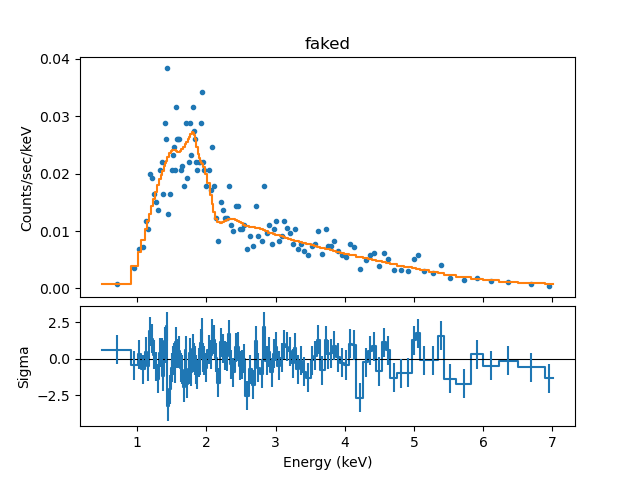### Figure 6: Plot of fit to simulated source-plus-background spectrum, with residuals

The plot may now be saved as a PostScript file:

sherpa> plt.savefig('simulation_fit_w_bkg.ps')


## Scripting It

The file fit.py is a Python script which performs the primary commands used above; it can be executed by typing %run -i fit.py on the Sherpa command line.

The Sherpa script command may be used to save everything typed on the command line in a Sherpa session:

sherpa> script(filename="sherpa.log", clobber=False)


(Note that restoring a Sherpa session from such a file could be problematic since it may include syntax errors, unwanted fitting trials, et cetera.)

## History

 02 Feb 2009 created for CIAO/Sherpa 4.1 29 Apr 2009 new script command is available with CIAO 4.1.2 12 Jan 2010 updated for CIAO 4.2 13 Jul 2010 updated for CIAO 4.2 Sherpa v2: removal of S-Lang version of thread. 15 Dec 2011 reviewed for CIAO 4.4: a work-around for a save_pha bug was added; response files used in examples updated for Chandra proposal cycle 14 13 Dec 2012 updated for CIAO 4.5: group commands no longer clear the existing data filter 04 Dec 2013 reviewed for CIAO 4.6: no changes 30 Jan 2015 updated for CY 17/CIAO 4.7 15 Dec 2015 updated for CY 18/CIAO 4.8 06 Dec 2016 updated for CY 19/CIAO 4.9/Python 3 23 Apr 2018 updated for CY 20/CIAO 4.10 13 Dec 2018 updated for CY 21/CIAO 4.11 11 Dec 2019 Updated for CIAO 4.12 by replacing print_window calls with the equivalent Matplotlib command plt.savefig. There have been no updates for the cycle 22 responses. 09 Mar 2020 changed reference of dataset ID faked to 1 for clarity. 17 Mar 2022 updated for CY 24/CIAO 4.14, updated figures with Matplotlib plots. 12 Dec 2022 updated for CY 25/CIAO 4.15, no content change.